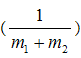Noblesville, IN

## Accident Reconstruction, Whiplash, Minor Vehicle Damage

(Part one)

First, the mass of each vehicle is relatively easy to measure. However, the cars are rarely, if ever, weighed, and usually the mass is determined by published data for each particular car model. This can be inaccurate, especially if one of the vehicles was carrying a heavy load or a number of passengers, or if the cars were modified in any way. So, immediately we have some uncertainty thrown into the analysis.

The second issue is v10, or the closing velocity of the crash. Without sophisticated equipment placed on both vehicles that would measure the speeds of each at the moment of impact, there is no way to accurately determine this number. Some accident reconstructionists simply use an estimate of what the witnesses state after the accident. A skilled engineer could examine the damage to the cars, and provide a rough estimate of the closing speed, but it would be an estimate of a range of possible speeds, and not a definitive speed. Furthermore, such analysis is seldom done on vehicles in low speed crashes. Frequently, the AR will simply begin with a low estimate of the crash speed, based upon what the at-fault driver states as the impact velocity.

Third, the coefficient of restitution also causes problems for the AR, because the only way to determine this number is to take identical cars, crash them together under conditions identical to the collision, and measure the amount of elasticity. This would be very expensive and impractical and is almost never done. The AR simply uses an estimate for this number that cannot be known for certain.

The last two portions of the equation—external forces and duration of collision—are not that important, as these variables are multiplied by a very small number:But again, these numbers are virtually impossible to determine. What are the external forces on the vehicles? To calculate this you would have to know whether the cars were on an incline, the condition of the road surface, whether either occupant hit the brakes, et cetera. There is simply no way to accurately measure these factors. As for the duration of the collision, or the Dt, a typical collision ranges from .1 to .2 seconds, but again, this is a range of values, and provides no certainty.

The bottom line is that we cannot calculate an accurate estimate of the Dv of the target vehicle without these important pieces of information. We might be able to estimate a range of possible Delta Vs after a careful analysis by an engineer, but this would still just be a range of values. (Δv—or the “change in” velocity—is important, because it is used to calculate acceleration; it is the rapid acceleration of the occupant that can cause injury in a crash.)

Despite the fact that you cannot accurately calculate Dv after a crash, many accident reconstructionists go even further, and provide a “calculation” of peak occupant acceleration. This is impossible. First, we don’t even know what the Delta v of the collision was, and because of that, we cannot calculate the acceleration of the target vehicle. Even if we did know the peak acceleration of the vehicle, however, we would still have no idea of the occupant acceleration, simply because of the huge number of variables that come into play (i.e., seat type, seat surface material, seat angle, occupant mass, occupant position, occupant gender, head position, et cetera).

The impossibility of accurately calculating occupant acceleration was clearly demonstrated by Siegmund et al. In this very well done study, the authors performed 39 test collisions with live occupants at 8 km/h (about 5 mph). In these carefully controlled tests—using the exact same vehicle, seat and test conditions for each crash—they found that the occupant G forces ranged from 6.7 to 12.0! That’s nearly a factor of two, in a carefully controlled environment. In the real world, these differences would be magnified to such a degree, that we couldn’t even begin to predict what the occupant forces might be.

In summary, understanding the basic math behind low speed crashes is important. The old maxim, “Garbage in, garbage out,” best sums up the problem of calculating accelerations after the fact. If there is no certainty of the inputs to the equation, the result of the equation is questionable at best.

1. Emori RI, Horiguchi J. Whiplash in low speed vehicle collisions. SAE 900542. 1990,103-108.
2. Seigmund GP, King DJ, Lawrence JM, et al. Head/neck kinematic response of human subjects in low-speed rear-end collisions. 41st Stapp Car Crash Conference, 1997. SAE 973341;357-385.